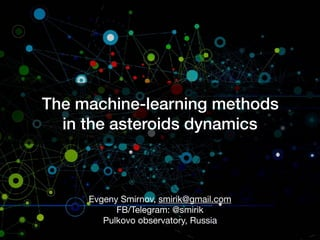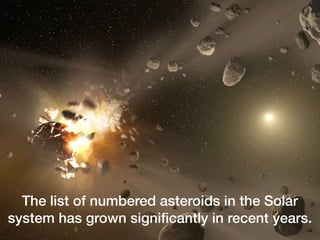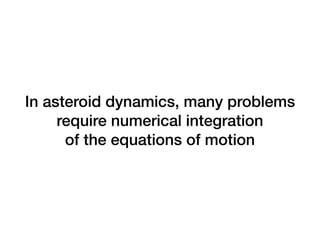Successfully reported this slideshow.

# A machine learning approach in the dynamics of asteroids×

# A machine learning approach in the dynamics of asteroids

In asteroid dynamics, many problems require numerical integration of the equations of motion. Due to the number of objects, it might require significant computational resources. Thus, one might find a better way to solve them — by using a machine learning approach.

In asteroid dynamics, many problems require numerical integration of the equations of motion. Due to the number of objects, it might require significant computational resources. Thus, one might find a better way to solve them — by using a machine learning approach.

### A machine learning approach in the dynamics of asteroids

1. 1. The machine-learning methods in the asteroids dynamics Evgeny Smirnov, smirik@gmail.com FB/Telegram: @smirik Pulkovo observatory, Russia
2. 2. The list of numbered asteroids in the Solar system has grown significantly in recent years.
3. 3. In asteroid dynamics, many problems require numerical integration   of the equations of motion
4. 4. This approach is   computationally expensive Therefore, fast, novel methods can be useful to work with big data
5. 5. AI & ML methods have become popular among the IT